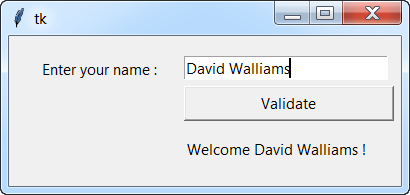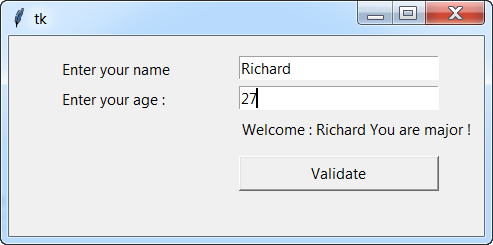## Pages

Python GUI Tkinter Exercises With Solutions

#### Exercise 194 || Solution

Write a program that asks the user to type  a word and return him its reverse without using any predefined function . Example if the user enters the word python, the program returns nohtyp to him as shown in the figure below:#### Exercise 195 || Solution

Create a graphical application in Python Tkinter that asks the user to enter an integer N and displays them the value of the sum "1 + 2 + ... + N" as shown in the figure below:#### Exercise 196 || Solution

Write a program in Python that displays a window to the user that asks them to enter an integer N and displays its factorial N !, as shown in the figure below:

#### Exercise 197 || Solution

Create a graphical application in Python Tkinter that asks the user to enter two integers and displays their sum as shown in the figure below:#### Exercise 198 || Solution

Create a graphical application with Python Tkinter which tests whether a given integer is a perfect square or not as shown in the figure below:#### Exercise 199 || Solution

Create a Python program using the Tkinter library, which displays a dialog box that ask user to type his name and display a dialog box asking him to enter his name and displays the welcome message followed by his name as shown in the figure below.#### Exercise 200 || Solution

Using the Tkinter Python library, write a Python program that displays a dialog box asking the user to enter an integer and return its double:

#### Exercise 201 || Solution

1. Write a program in Python that lists all divisors of a given integer N.
2. Create a program in Python that which a Tkinter window asking the user to enter an integer N and returns all the divisors of N.#### Exercise 202  ||  Solution

Create a Tkinter GUI interface, which calculates the greatest common divisor GCD and the least common multiple LCM graphically as shown in the figure below:

#### Exercise 203

Using the Tkinter Python library, write a Python program that displays a dialog asking the user to enter their name and their age and return the message as showing in the figure below:
- Hello followed by his name
- You are major ! age if the age entered is > = 18
- You are minor ! if the age entered is > 18Younes   Derfoufi
www.my-courses.net

## Python Exercises With Solutions

1. Python Arithmetic Exercises
2. Equations & System Of Equations
3. Polynomials and Functions
4. Python Arithmetic Exercises
5. Numpy, Matplolib, Sympy , Scipy - Exercises
6. Python Arithmetic Exercises

Younes Derfoufi

## Sample Text